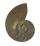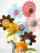# Eqn

Solve equation with fractions:

2x/3-50=40+x/4

x =  216

### Step-by-step explanation:

2x/3-50=40+x/4

2•x/3-50=40+x/4

5x = 1080

x = 216

Our simple equation calculator calculates it.Did you find an error or inaccuracy? Feel free to write us. Thank you!Tips to related online calculators
Need help to calculate sum, simplify or multiply fractions? Try our fraction calculator.
Do you have a linear equation or system of equations and looking for its solution? Or do you have a quadratic equation?

## Related math problems and questions:

• Equation with fractionsSolve an equation: 1- (2x-5)/(6) =3- (x)/(4) It is an equation with fractions.
• Eq-fracSolve the following equation with fractions: h + 1/3 =5/3
• Equation with xSolve the following equation: 2x- (8x + 1) - (x + 2) / 5 = 9
• Equation 15Solve equation with variables on both sides:
• Simple equation 324 = n • 27, solve for n
• EquationSolve equation and check the result: 1.4x - 3/2 + x - 9,8 = x + 0,4/3 - 7 + 1,6/6
• Over fractionSolve equation: p over 5 equals fraction numerator 7 p over denominator 6 end fraction plus 5
• Find xSolve: if 2(x-1)=14, then x= (solve an equation with one unknown)
• Reciprocal equation 3Solve reciprocal equation: 1/2 + 2/3=1/x
• Fraction + eqSolve following simple equation with fractions: -5/6(8+5b) = 75 + 5/3b
• InqualitySolve inequality: 3x + 6 > 14
• Simple equation 5Solve equation with fractions: X × 3/8 = 1/2
• Equation 11Solve equation: 0=y-1,2.(y-1,5)
• Logarithmic equationSolve equation: log13(7x + 12) = 0
• SolveSolve this direct relation by the triplet: 0.25h. .. . . .. .. . . .. .. .. 3 pcs 6.5h. .. . . .. .. . . .. .. . . .. .. x pcs
• Equation 25Solve following simple equation: 3/4(x+5)=1/2(x+9)
• Simple equation 6Solve equation with one variable: X/2+X/3+X/4=X+4